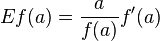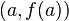Tags
#calculus #elasticity #has-images #mathematics
Question

In mathematics, the elasticity or point elasticity of a positive differentiable function f of a positive variable (positive input, positive output) at point a is defined as using functions and their derivativesmeaning in words: [...]

It is thus the ratio of the relative (percentage) change in the function's output $$f(a)$$ with respect to the relative change in its input $$a$$, for infinitesimal changes from a point.

Tags
#calculus #elasticity #has-images #mathematics
Question

In mathematics, the elasticity or point elasticity of a positive differentiable function f of a positive variable (positive input, positive output) at point a is defined as using functions and their derivativesmeaning in words: [...]

?

Tags
#calculus #elasticity #has-images #mathematics
Question

In mathematics, the elasticity or point elasticity of a positive differentiable function f of a positive variable (positive input, positive output) at point a is defined as using functions and their derivativesmeaning in words: [...]

It is thus the ratio of the relative (percentage) change in the function's output $$f(a)$$ with respect to the relative change in its input $$a$$, for infinitesimal changes from a point.
If you want to change selection, open original toplevel document below and click on "Move attachment"

#### Parent (intermediate) annotation

Open it
the elasticity or point elasticity of a positive differentiable function f of a positive variable (positive input, positive output) at point a is defined as using functions and their derivatives meaning in words: <span>It is thus the ratio of the relative (percentage) change in the function's output $$f(a)$$ with respect to the relative change in its input $$a$$, for infinitesimal changes from a point . <span><body><html>

#### Original toplevel document

Elasticity of a function - Wikipedia, the free encyclopedia
er:filter:minify-css:7:3904d24a08aa08f6a68dc338f9be277e */ Elasticity of a function From Wikipedia, the free encyclopedia Jump to: navigation, search <span>In mathematics, the elasticity or point elasticity of a positive differentiable function f of a positive variable (positive input, positive output) at point a is defined as or equivalently It is thus the ratio of the relative (percentage) change in the function's output with respect to the relative change in its input , for infinitesimal changes from a point . Equivalently, it is the ratio of the infinitesimal change of the logarithm of a function with respect to the infinitesimal change of the logarithm of the argument. The elasticity of a function is a constant if and only if the function has the form for a constant . The elasticity at a point is the limit of the arc elasticity between two points as

#### Summary

status measured difficulty not learned 37% [default] 0

No repetitions